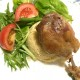# Category Theory 9: Natural Transformations, BiCategoriesMath Online Tom Circle

In essence, in all kinds of Math, we do 3 things:

1) Find Pattern among objects (numbers, shapes, …),
2) Operate inside the objects (+ – × / …),
3) Swap the object without modifying it (rotate, flip, move around, exchange…).

Category consists of :
1) Find pattern thru Universal Construction in Objects (Set, Group, Ring, Vector Space, anything )
2) Functor which operates on 1).
3) Natural Transformation as in 3).

\$latex boxed {text {Natural Transformation}}&fg=000000&s=3\$
\$latex Updownarrow \$

\$latex boxed {text {Morphism of Functors}}&fg=aa0000&s=3\$Analogy:

Functors (F, G) :=operation inside a container
\$latex boxed { F :: X to F_{X}, : F :: Y to F_{Y}}&fg=0000aa&s=3\$

\$latex boxed {G :: X to G_{X}, : G :: Y to G_{Y}}&fg=00aa00&s=3\$

Natural Transformation (\$latex {eta_{X}, eta_{Y}}&fg=ee0000&s=3\$) := swap the content ( \$latex F_{X} text { with } G_{X}, F_{Y} text { with } G_{Y} \$) in the…

View original post 105 more words## Author: tomcircle

Math amateur

Posted on Categories math

This site uses Akismet to reduce spam. Learn how your comment data is processed.